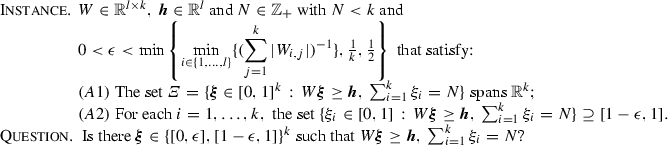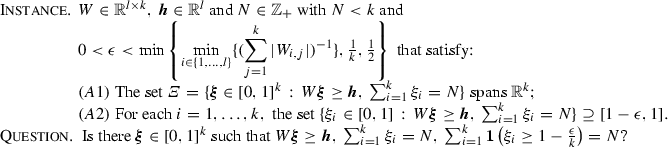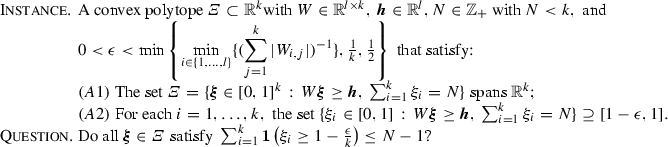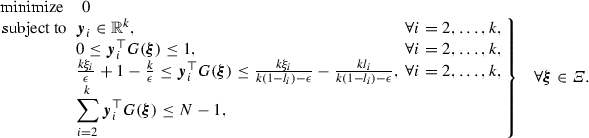# Binary decision rules for multistage adaptive mixed-integer optimization

## Abstract

Decision rules provide a flexible toolbox for solving computationally demanding, multistage adaptive optimization problems. There is a plethora of real-valued decision rules that are highly scalable and achieve good quality solutions. On the other hand, existing binary decision rule structures tend to produce good quality solutions at the expense of limited scalability and are typically confined to worst-case optimization problems. To address these issues, we first propose a linearly parameterised binary decision rule structure and derive the exact reformulation of the decision rule problem. In the cases where the resulting optimization problem grows exponentially with respect to the problem data, we provide a systematic methodology that trades-off scalability and optimality, resulting to practical binary decision rules. We also apply the proposed binary decision rules to the class of problems with random-recourse and show that they share similar complexity as the fixed-recourse problems. Our numerical results demonstrate the effectiveness of the proposed binary decision rules and show that they are (i) highly scalable and (ii) provide high quality solutions.

This is a preview of subscription content, access via your institution.

## References

1. Anderson, B.D.O., Moore, J.B.: Optimal Control: Linear Quadratic Methods. Prentice Hall, Upper Saddle River (1990)

2. Bampou, D., Kuhn, D.: Scenario-free stochastic programming with polynomial decision rules. In: 50th IEEE Conference on Decision and Control and European Control Conference, Orlando, FL, USA, pp. 7806–7812 (December 2011)

3. Ben-Ta, A., den Hertog, D.: Immunizing Conic Quadratic Optimization Problems Against Implementation Errors, vol. 2011-060. Tilburg University, Tilburg (2011)

4. Ben-Tal, A., El Ghaoui, L., Nemirovski, A.: Robust Optimization. Princeton University Press, Princeton (2009)

5. Ben-Tal, A., Golany, B., Shtern, S.: Robust multi-echelon multi-period inventory control. Eur. J. Oper. Res. 199(3), 922–935 (2009)

6. Ben-Tal, A., Goryashko, A., Guslitzer, E., Nemirovski, A.: Adjustable robust solutions of uncertain linear programs. Math. Program. 99(2), 351–376 (2004)

7. Ben-Tal, A., Nemirovski, A.: Robust convex optimization. Math. Oper. Res. 23(4), 769–805 (1998)

8. Bertsimas, D., Caramanis, C.: Adaptability via sampling. In: 46th IEEE Conference on Decision and Control, New Orleans, LA, USA, pp. 4717–4722 (December 2007)

9. Bertsimas, D., Caramanis, C.: Finite adaptability in multistage linear optimization. IEEE Trans. Autom. Control 55(12), 2751–2766 (2010)

10. Bertsimas, D., Georghiou, A.: Design of near optimal decision rules in multistage adaptive mixed-integer optimization. Oper. Res. 63(3), 610–627 (2015)

11. Bertsimas, D., Iancu, D.A., Parrilo, P.A.: Optimality of affine policies in multi-stage robust optimization. Math. Oper. Res. 35(2), 363–394 (2010)

12. Bertsimas, D., Iancu, D.A., Parrilo, P.A.: A hierarchy of near-optimal policies for multistage adaptive optimization. IEEE Trans. Autom. Control 56(12), 2809–2824 (2011)

13. Calafiore, G.C.: Multi-period portfolio optimization with linear control policies. Automatica 44(10), 2463–2473 (2008)

14. Calafiore, G.C.: An affine control method for optimal dynamic asset allocation with transaction costs. SIAM J. Control Optim. 48(4), 2254–2274 (2009)

15. Caramanis, C.: Adaptable Optimization: Theory and Algorithms. Ph.D. thesis, Massachusetts Institute of Technology (2006)

16. Chen, X., Sim, M., Sun, P., Zhang, J.: A linear decision-based approximation approach to stochastic programming. Oper. Res. 56(2), 344–357 (2008)

17. Chen, X., Zhang, Y.: Uncertain linear programs: extended affinely adjustable robust counterparts. Oper. Res. 57(6), 1469–1482 (2009)

18. Dyer, M., Stougie, L.: Computational complexity of stochastic programming problems. Math. Program. 106(3), 423–432 (2006)

19. Garstka, S.J., Wets, R.J.-B.: On decision rules in stochastic programming. Math. Program. 7(1), 117–143 (1974)

20. Georghiou, A., Wiesemann, W., Kuhn, D.: Generalized decision rule approximations for stochastic programming via liftings. Math. Program. 152(1–2), 301–338 (2015)

21. Goh, J., Sim, M.: Distributionally robust optimization and its tractable approximations. Oper. Res. 58(4), 902–917 (2010)

22. Goulart, P.J., Kerrigan, E.C., Maciejowski, J.M.: Optimization over state feedback policies for robust control with constraints. Automatica 42(4), 523–533 (2006)

23. Gounaris, C.E., Wiesemann, W., Floudas, C.A.: The robust capacitated vehicle routing problem under demand uncertainty. Oper. Res. 61(3), 677–693 (2013)

24. Hanasusanto, G.A., Kuhn, D., Wiesemann, W.: K-Adaptability in Two-Stage Robust Binary Programming. Oper Res. 63(4), 877–891 (2015). doi:10.1287/opre.2015.1392

25. Hanasusanto, G.A., Roitch, V., Kuhn, D., Wiesemann, W.: Ambiguous joint chance constraints under mean and dispersion information. Submitted for publication, available via Optimization-Online (2016)

26. Kuhn, D., Wiesemann, W., Georghiou, A.: Primal and dual linear decision rules in stochastic and robust optimization. Math. Program. 130(1), 177–209 (2011)

27. Papadimitriou, C.H.: On the complexity of integer programming. J. Assoc. Comput. Mach. 28(4), 765–768 (1981)

28. Rockafellar, R.T., Wets, R.J.B.: Variational Analysis. Springer, Dordrecht (1997)

29. See, C.-T., Sim, M.: Robust approximation to multiperiod inventory management. Oper. Res. 58(3), 583–594 (2010)

30. Shapiro, A., Nemirovski, A.: On complexity of stochastic programming problems. Appl. Optim. 99(1), 111–146 (2005)

31. Skaf, J., Boyd, S.P.: Design of affine controllers via convex optimization. IEEE Trans. Autom. Control 55(11), 2476–2487 (2010)

## Acknowledgements

We like to thank Dr. Wolfram Wiesemann for very helpful discussions, and the Associate Editor and referees of the paper for their suggestions which improved the paper significantly.

## Author information

Authors

### Corresponding author

Correspondence to Dimitris Bertsimas.

## Appendix: Technical proofs

### Appendix: Technical proofs

In this section, we provide the proof for Theorem 1. To do this, we need the following auxiliary results given in Lemmas 1, 2 and 3. Lemma 1 gives the complexity of the epsilon integer feasibility problem, which will be used in Lemmas 2 and 3 to prove that checking feasibility of a semi-infinite constraint involving indicator functions is $$\mathrm {NP}$$-hard.

### Lemma 1

(Epsilon integer feasibility problem) The following decision problem is $$\mathrm {NP}$$-hard:(7.1)

The condition $$\varvec{\xi } \in \{[0,\epsilon ],[1-\epsilon ,1]\}^k$$ implies that each component of $$\varvec{\xi }$$ can take values either in the set $$[0,\epsilon ]$$ or in the set $$[1-\epsilon ,1]$$. Note that (A1) is a standard assumption, while (A2) is non-restrictive since if the projection of a component of $$\varvec{\xi }$$ is strictly less than $$1-\epsilon$$, (or strictly greater than $$\epsilon$$) then that component of $$\varvec{\xi }$$ can be a priori fixed to an element of $$[0,\epsilon ]$$ (or $$[1-\epsilon ]$$), or in the case where $$\epsilon< \xi _i < 1-\epsilon$$ the dimension of $$\varXi$$ can be reduced without changing the problem structure. Assumptions (A1) and (A2) are not necessary for the proofs of Lemmas 1, 2 and 3, but will be used in the proof of Theorem 1.

### Proof

The proof is a slight variant of [25, Lemma 2] and references within.$$\square$$

In particular, the work of Hanasusanto et al.  shows that the epsilon integer feasibility problem is in fact equivalent to the integer feasibility problem which is known to be $$\mathrm {NP}$$-hard, see . More precisely, they prove that the decision problem (7.1) has an affirmative answer if and only if there exists a vector $$\varvec{\chi }\in \{0,1\}^k$$ which gives an affirmative answer to the corresponding integer feasibility problem, with $$\chi _i=1$$ if $$\xi _i>1-\epsilon$$, and $$\chi _i=0$$ otherwise.

### Lemma 2

The following decision problem is $$\mathrm {NP}$$-hard:(7.2)

### Proof

We will prove the assertion in two steps. In the first step, assume that the decision problem (7.1) has an affirmative answer, i.e., there exists a $$\varvec{\xi } \in \{[0,\epsilon ],[1-\epsilon ,1]\}^k$$ that satisfies (7.1). Then, by [25, Lemma 2] there exists $$\varvec{\chi }\in \{0,1\}^k$$ with $$\chi _i=1$$ if $$\xi _i>1-\epsilon$$, and $$\chi _i=0$$ otherwise, that satisfies constraints $$W\varvec{\chi }\ge \varvec{h},\,\sum _{i=1}^k \chi _i = N$$. It is easy to see that this vector $$\varvec{\chi }$$ will also satisfy $$\sum _{i=1}^k \varvec{1}\left( \chi _i \ge 1 - \frac{\epsilon }{k}\right) = N$$ due to the fact that $$N<k$$, and therefore decision problem (7.2) will have an affirmative answer. For the second step, assume that the decision problem (7.2) has an affirmative answer. We now prove by contradiction that a vector $$\varvec{\xi } \in [0,1]^k$$ satisfying (7.2) must also satisfy (7.1). Assume that there exists $$\varvec{\xi }$$ with $$\epsilon<\xi _1<1-\epsilon$$ that satisfies $$W\varvec{\xi }\ge \varvec{h},\,\sum _{i=1}^k \xi _i = N,\, \sum _{i = 1}^k \varvec{1}\left( \xi _i \ge 1 - \frac{\epsilon }{k}\right) =N$$. Since $$\epsilon<\xi _1<1-\epsilon$$, then $$\sum _{i = 2}^k \xi _i < N - \epsilon$$ and as a result $$\sum _{i = 2}^k \varvec{1}(\xi _i \ge 1 - \epsilon /k) < N$$, which contradicts the assumption that $$\sum _{i = 1}^k \varvec{1}\left( \xi _i \ge 1 - \frac{\epsilon }{k}\right) = N$$. The latter follows since if distributed evenly, the value each $$\xi _i$$ can take is $$\xi _i = 1-\frac{\epsilon }{N}$$, for $$i=2,\ldots ,N+1$$, and since $$N<k$$ it implies that $$\frac{1}{N}(N-\epsilon ) = 1-\frac{\epsilon }{N} < 1-\frac{\epsilon }{k}$$.

Therefore, we conclude that $$\varvec{\xi } \in \{[0,\epsilon ],[1-\epsilon ,1]\}^k$$. Together, the two steps allow us to conclude that (7.2) is $$\mathrm {NP}$$-hard. $$\square$$

The following lemma provides the key ingredient for proving Theorem 1.

### Lemma 3

The following decision problem is $$\mathrm {NP}$$-hard:(7.3)

### Proof

Notice that the decision problem (7.3) is the negation of the decision problem (7.2). In other words, the decision problem (7.3) evaluates to true if there is no $$\varvec{\xi }$$ such that

\begin{aligned} \varvec{\xi } \in [0,1]^k\text { such that }W\varvec{\xi }\ge \varvec{h},\,\sum _{i=1}^k \xi _i = N,\, \sum _{i = 1}^k \varvec{1}\left( \xi _i \ge 1 - \frac{\epsilon }{k}\right) =N, \end{aligned}

which corresponds to checking if decision problem (7.2) holds. Notice that there is no $$\varvec{\xi }$$ such that $$\sum _{i = 1}^k \varvec{1}\left( \xi _i \ge 1 - \frac{\epsilon }{k}\right) > N$$. This is the case since $$\sum _{i=1}^k \xi _i = N$$ and $$N<k$$, implying that $$N - N(1-\epsilon /k) = N\epsilon /k < 1$$. The reverse statement holds in a similar manner.$$\square$$

We now have all the ingredients to prove Theorem 1.

### Proof of Theorem 1

Let $$\varXi$$ be a convex polytope given by

\begin{aligned} \varXi :=\left\{ \varvec{\xi }\in [0,1]^k\,:\,\xi _1 = 1,\, W\varvec{\xi }\ge \varvec{h},\;\;\sum _{i=2}^k \xi _i= N\right\} , \end{aligned}
(7.4)

with $$N\in \mathbb {Z}_+$$, $$N < k-1$$, and the choice of W and $$\varvec{h}$$ are such that $$\varXi$$ spans $${\mathbb R}^k$$ and the projection of $$\varXi$$ on each component $$\xi _i$$ is a superset of $$[1-\epsilon ,1]$$ for $$i = 2,\ldots ,k$$, with fixed $$\epsilon \in (0,\min \{{\min _{i\in \{1,\ldots ,l\}}\{(\sum _{j=1}^k |W_{i,j}|)^{-1}\}},\frac{1}{k},\frac{1}{2}\})$$. In other words, the set $$\varXi$$ satisfies (A1) and (A2) in the decision problem (7.3).

Given the above instance, we can construct the projection of $$\varXi$$ on each component $$\xi _i$$, i.e, for each $$i = 2,\ldots ,k$$, there exists $$l_i\in [0,1-\epsilon ]$$ such that $$\{\xi _i\in [0,1]\,:\, W\varvec{\xi }\ge \varvec{h},\,\sum _{i=1}^k \xi _i = N\} = [l_i,1]$$. Now, let $$\varvec{\alpha }_i = \varvec{e}_i$$ and $$\beta _i=1 - \frac{\epsilon }{k}$$, for $$i=2,\ldots ,k$$ in the description of $$G(\cdot )$$ in (2.4b). By construction, the components of $$G(\varvec{\xi })$$ are linearly independent for all $$\varvec{\xi }\in \varXi$$, i.e,

\begin{aligned} \varvec{v}^\top G(\varvec{\xi }) = 0, \quad \forall \varvec{\xi }\in \varXi \quad \implies \quad \varvec{v} = 0. \end{aligned}

This is the case since each component of $$G(\varvec{\xi })$$ is non-constant on disjoint subsets of $${\mathbb R}^k$$ and each of these subsets has a non-empty intersection with $$\varXi$$, with only $$G_1(\varvec{\xi })=1$$ being constant for all $$\varvec{\xi }\in \varXi$$. This is satisfied by the construction of $$\varXi$$ which is a convex set, spans $${\mathbb R}^k$$ and the projection of $$\varXi$$ on each component $$\xi _i$$ is $$[l_i,1]$$ for $$i = 2,\ldots ,k$$, with $$l_i \le 1-\epsilon< 1- \frac{\epsilon }{k} < 1$$.

We define the following feasibility problem:(7.5)

It is easy to see that for each $$i=2,\ldots ,k$$, a feasible vector $$\varvec{y}_i$$ in the second and third constraints satisfies $$\varvec{e}_i \le \varvec{y}_i \le \varvec{e}_i + \varvec{v}_i$$, for some $$\varvec{v}_i\in {\mathbb R}^k$$ with $$v_{i,i} = 0$$. In particular, the solution $$\varvec{y}_i=\varvec{e}_i$$ for each $$i=2,\ldots ,k$$ is feasible in the second and third constraint. This is the case since for each i, the second and third constraint create the convex hull of function $$\varvec{1}\left( \xi _i \ge 1 - \frac{\epsilon }{k}\right)$$ on $$\xi _i\in [l_i,1]$$, see Fig. 7.

We will also show that for the smallest N for which the last constraint is feasible, the only feasible solution is $$\varvec{y}_i = \varvec{e}_i$$ for all $$i=2,\ldots ,k$$. We will show this by contradiction. Assume that for each $$i=2,\ldots ,k$$, there exist non-zero vectors $$\varvec{v}_i\in {\mathbb R}^k$$ such that $$\varvec{y}_i = \varvec{e}_i + \varvec{v}_i$$ is feasible. Since we have the smallest N, with $$N<k-1$$, constraint

\begin{aligned} \displaystyle \sum _{i=2}^k (\varvec{e}_i + \varvec{v}_i)^\top G(\varvec{\xi })\le N-1, \quad \forall \xi \in \varXi , \end{aligned}

implies that $$\varvec{v}_i^\top G(\varvec{\xi }) = 0$$ for all $$\varvec{\xi }\in \varXi$$ and $$i=2,\ldots ,k$$. This contradicts the fact that the components of the components of $$G(\varvec{\xi })$$ are linearly independent for all $$\varvec{\xi }\in \varXi$$.

Therefore, we conclude that if Problem (7.5) is feasible, then $$\varvec{y}_i = \varvec{e}_i$$ for all $$i=2,\ldots ,k$$ is a feasible solution that satisfies the last constraint, and in addition, if constraint $$\sum _{i = 2}^k \varvec{1}\left( \xi _i \ge 1 - \frac{\epsilon }{k}\right) \le N-1$$ is feasible, then Problem (7.5) is feasible, i.e.,

\begin{aligned} \sum _{i = 2}^k \varvec{1}\left( \xi _i \ge 1 - \frac{\epsilon }{k}\right) \le N-1, \quad \forall \varvec{\xi } \in \varXi \quad \iff \quad \text {Problem }(A.6.2)\text { is feasible}. \end{aligned}

Hence, Lemma 3 implies that checking the feasibility of Problem (7.5) is $$\mathrm {NP}$$-hard. Since the admissible feasible solutions are integers, i.e., $$\varvec{y_i}\in \mathbb {Z}^{k}$$ for all $$i=2,\ldots ,k$$, Problem (7.5) can be reduced to an instance of Problem (2.10). Therefore,Lemma 3 implies that Problems (2.10) and (2.14) are $$\mathrm {NP}$$-hard, even when $$Y\in \mathbb {Z}^{k\times g}$$ is relaxed to $$Y\in {\mathbb R}^{k\times g}$$.$$\square$$

## Rights and permissions

Reprints and Permissions